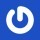^ 回到顶部
• 人生没有定律，每个人都有自己的节奏
• 本站wordpress建站教程均通过实践后发布，希望对你有帮助，如果有代码出错，请联系站长解决
• 希望你的坚持，都是因为热爱，而不是因为不甘心
• 8年wordpress建站经验，5星服务品质
• 那些不愿意让你吃亏的人，才是真正值得你深交的人，也是值得你付出时间的人
• 阿里云ECS服务器

# 零起点php入门第12课-php数组（1）

1、数组的优势

```<?php
\$stu01=67;					//声明多个变量存储学生成绩
\$stu02=77;
\$stu03=96;
...
\$stun=100;
\$average=(\$stu01+\$stu02+stu03+...+\$stun)/n;			//求取平均值
?>
```

```<?php
\$stu=array(67,77,96,...,100);			//定义一个数组
\$total=array_sum(\$stu);			//求取总成绩
\$average=\$total/n;			//求取平均值
?>
```

2、定义数组

PHP 中定义数组使用的是 array 结构，它的语法形式如下所示：

array array ([mixed values ]… )

\$arr=array(98,’hello’,67,’A’,85,NULL);

3、访问数组元素

```<?php
\$arr=array(98,'hello',67,'A',85,NULL);		//定义一个数组
echo "输出第一个元素：{\$arr}";			//输出数组的元素
echo "<br />输出第二个元素：{\$arr}";
echo "<br />输出第三个元素：{\$arr}";
echo "<br />输出第四个元素：{\$arr}";
echo "<br />输出第五个元素：{\$arr}";
echo "<br />输出第六个元素：{\$arr}";
?>```

```输出第一个元素：98

```<?php
\$arr=array(98,'hello',67,'A',85,NULL);		//定义一个数组
\$x=0;			//定义三个作为下标的变量
\$y=3;
\$z=5;
echo "下标为{\$x}的元素为{\$arr[\$x]}。<br />";		//输出对应下标及元素值
echo "下标为{\$y}的元素为{\$arr[\$y]}。<br />";
echo "下标为{\$z}的元素为{\$arr[\$z]}。<br />";
\$x=2;			//为变量 x 重新赋值
echo "下标为{\$x}的元素为{\$arr[\$x]}。";		//输出对应下标及元素值
?>```

```<?php
function num(){			//定义函数
\$x=7;
\$y=6;
return \$x-\$y;			//返回值为 7
}
\$arr=array(98,'hello',67,'A',85,NULL);		//定义一个数组
\$a=1;					//定义两个变量
\$b=3;
echo '数组下标为 4 的元素为：'.\$arr[\$a+\$b];		//表达式作为下标
echo '<br />数组下标为 1 的元素为：'.\$arr[num()];		//表达式作为下标
?>```### 如有疑问，请前往问答中心反馈！

1.唯历史
收藏了，我也学习一下
2. 陌小雨网站管理员
签到成功！签到时间：下午6:46:50，陌小雨，你很棒！一起加油哦哦~
3.跨境电商
很好，谢谢分享。：P